23

# Pressure & Gas Laws

Gases exert pressure, which is force per unit area. The pressure of a gas may be expressed in the SI unit of pascal or kilopascal, as well as in many other units including torr, atmosphere, and bar. Atmospheric pressure is measured using a barometer; other gas pressures can be measured using one of several types of manometers. The behavior of gases can be described by several laws based on experimental observations of their properties. The pressure of a given amount of gas is directly proportional to its absolute temperature, provided that the volume does not change (Amontons’s law). The volume of a given gas sample is directly proportional to its absolute temperature at constant pressure (Charles’s law). The volume of a given amount of gas is inversely proportional to its pressure when temperature is held constant (Boyle’s law). Under the same conditions of temperature and pressure, equal volumes of all gases contain the same number of molecules (Avogadro’s law).

## 23.1 Gas Pressure

### Learning Objectives

By the end of this section, you will be able to:

• Define the property of pressure
• Define and convert among the units of pressure measurements
• Describe the operation of common tools for measuring gas pressure
• Calculate pressure from manometer data

The earth’s atmosphere exerts a pressure, as does any other gas. Although we do not normally notice atmospheric pressure, we are sensitive to pressure changes—for example, when your ears “pop” during take-off and landing while flying, or when you dive underwater. Gas pressure is caused by the force exerted by gas molecules colliding with the surfaces of objects (Figure 23.1). Although the force of each collision is very small, any surface of appreciable area experiences a large number of collisions in a short time, which can result in a high pressure. In fact, normal air pressure is strong enough to crush a metal container when not balanced by equal pressure from inside the container.

Figure 23.1

The atmosphere above us exerts a large pressure on objects at the surface of the earth, roughly equal to the weight of a bowling ball pressing on an area the size of a human thumbnail.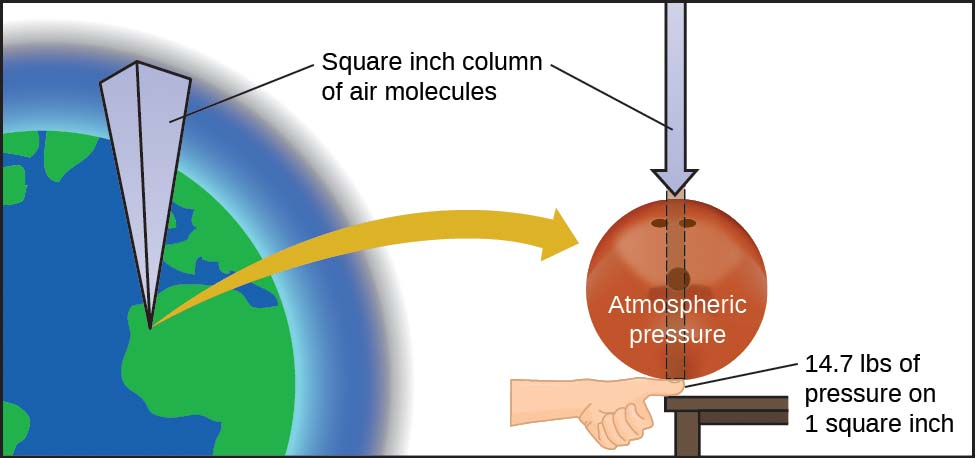Atmospheric pressure is caused by the weight of the column of air molecules in the atmosphere above an object, such as the tanker car. At sea level, this pressure is roughly the same as that exerted by a full-grown African elephant standing on a doormat, or a typical bowling ball resting on your thumbnail. These may seem like huge amounts, and they are, but life on earth has evolved under such atmospheric pressure. If you actually perch a bowling ball on your thumbnail, the pressure experienced is twice the usual pressure, and the sensation is unpleasant.

In general, pressure is defined as the force exerted on a given area: P=FA.P=FA. Note that pressure is directly proportional to force and inversely proportional to area. Thus, pressure can be increased either by increasing the amount of force or by decreasing the area over which it is applied; pressure can be decreased by decreasing the force or increasing the area.

Let’s apply this concept to determine which exerts a greater pressure in Figure 23.2—the elephant or the figure skater? A large African elephant can weigh 7 tons, supported on four feet, each with a diameter of about 1.5 ft (footprint area of 250 in2), so the pressure exerted by each foot is about 14 lb/in2:

pressure per elephant foot=14,000lbelephant×1 elephant4 feet×1 foot250in2=14lb/in2pressure per elephant foot=14,000lbelephant×1 elephant4 feet×1 foot250in2=14lb/in2

The figure skater weighs about 120 lbs, supported on two skate blades, each with an area of about 2 in2, so the pressure exerted by each blade is about 30 lb/in2:

Even though the elephant is more than one hundred-times heavier than the skater, it exerts less than one-half of the pressure. On the other hand, if the skater removes their skates and stands with bare feet (or regular footwear) on the ice, the larger area over which their weight is applied greatly reduces the pressure exerted:

pressure per human foot=120lbskater×1 skater2 feet×1 foot30in2=2lb/in2pressure per human foot=120lbskater×1 skater2 feet×1 foot30in2=2lb/in2

Figure 23.2

Although (a) an elephant’s weight is large, creating a very large force on the ground, (b) the figure skater exerts a much higher pressure on the ice due to the small surface area of the skates. (credit a: modification of work by Guido da Rozze; credit b: modification of work by Ryosuke Yagi)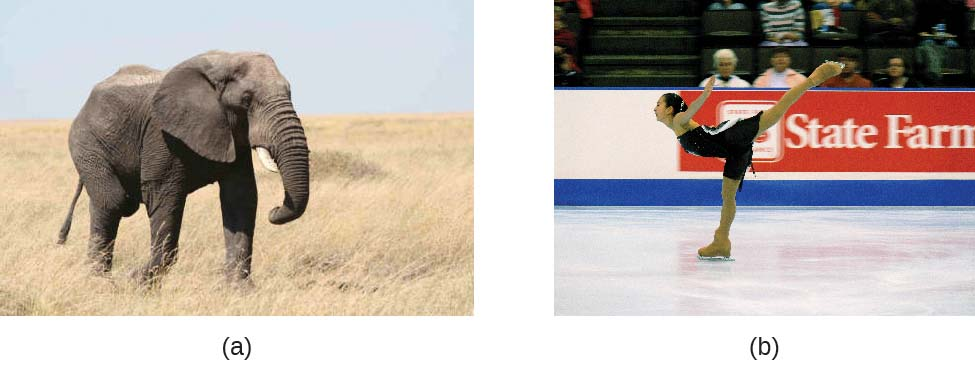The SI unit of pressure is the pascal (Pa), with 1 Pa = 1 N/m2, where N is the newton, a unit of force defined as 1 kg m/s2. One pascal is a small pressure; in many cases, it is more convenient to use units of kilopascal (1 kPa = 1000 Pa) or bar (1 bar = 100,000 Pa). In the United States, pressure is often measured in pounds of force on an area of one square inch—pounds per square inch (psi)—for example, in car tires. Pressure can also be measured using the unit atmosphere (atm), which originally represented the average sea level air pressure at the approximate latitude of Paris (45°). Table 23.1 provides some information on these and a few other common units for pressure measurements

Table 23.1

Pressure Units

Unit Name and AbbreviationDefinition or Relation to Other Unit
pascal (Pa)1 Pa = 1 N/m2
recommended IUPAC unit
kilopascal (kPa)1 kPa = 1000 Pa
pounds per square inch (psi)air pressure at sea level is ~14.7 psi
atmosphere (atm)1 atm = 101,325 Pa = 760 torr
air pressure at sea level is ~1 atm
bar (bar, or b)1 bar = 100,000 Pa (exactly)
commonly used in meteorology
millibar (mbar, or mb)1000 mbar = 1 bar
inches of mercury (in. Hg)1 in. Hg = 3386 Pa
used by aviation industry, also some weather reports
torr1 torr=1760atm1 torr=1760atm
named after Evangelista Torricelli, inventor of the barometer
millimeters of mercury (mm Hg)1 mm Hg ~1 torr

### Example 23.1

#### Conversion of Pressure Units

The United States National Weather Service reports pressure in both inches of Hg and millibars. Convert a pressure of 29.2 in. Hg into:

(a) torr

(b) atm

(c) kPa

(d) mbar

#### Solution

This is a unit conversion problem. The relationships between the various pressure units are given in Table 23.1.

(a) 29.2in Hg×25.4mm1in×1 torr1mm Hg=742 torr29.2in Hg×25.4mm1in×1 torr1mm Hg=742 torr

(b) 742torr×1 atm760torr=0.976 atm742torr×1 atm760torr=0.976 atm

(c) 742torr×101.325 kPa760torr=98.9 kPa742torr×101.325 kPa760torr=98.9 kPa

(d) 98.9kPa×1000Pa1kPa×1bar100,000Pa×1000 mbar1bar=989 mbar98.9kPa×1000Pa1kPa×1bar100,000Pa×1000 mbar1bar=989 mbar

A typical barometric pressure in Kansas City is 740 torr. What is this pressure in atmospheres, in millimeters of mercury, in kilopascals, and in bar?

0.974 atm; 740 mm Hg; 98.7 kPa; 0.987 bar

We can measure atmospheric pressure, the force exerted by the atmosphere on the earth’s surface, with a barometer (Figure 23.3). A barometer is a glass tube that is closed at one end, filled with a nonvolatile liquid such as mercury, and then inverted and immersed in a container of that liquid. The atmosphere exerts pressure on the liquid outside the tube, the column of liquid exerts pressure inside the tube, and the pressure at the liquid surface is the same inside and outside the tube. The height of the liquid in the tube is therefore proportional to the pressure exerted by the atmosphere.

Figure 23.3

In a barometer, the height, h, of the column of liquid is used as a measurement of the air pressure. Using very dense liquid mercury (left) permits the construction of reasonably sized barometers, whereas using water (right) would require a barometer more than 30 feet tall.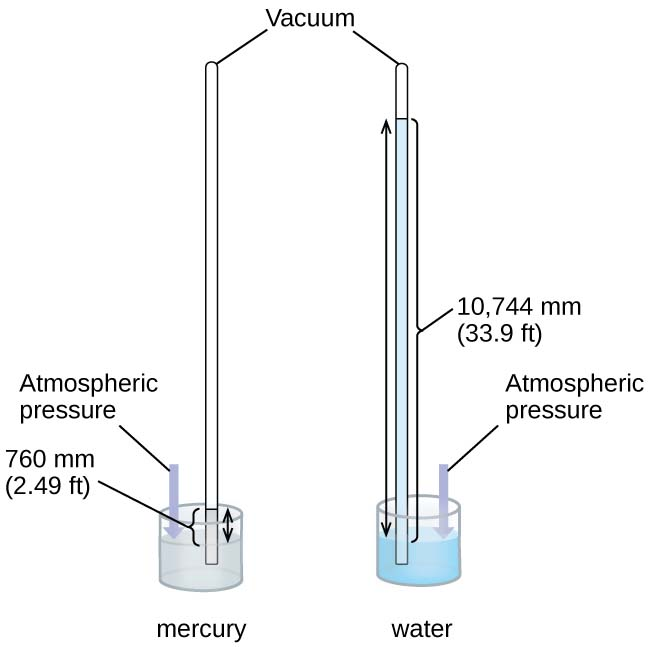If the liquid is water, normal atmospheric pressure will support a column of water over 10 meters high, which is rather inconvenient for making (and reading) a barometer. Because mercury (Hg) is about 13.6-times denser than water, a mercury barometer only needs to be 113.6113.6 as tall as a water barometer—a more suitable size. Standard atmospheric pressure of 1 atm at sea level (101,325 Pa) corresponds to a column of mercury that is about 760 mm (29.92 in.) high. The torr was originally intended to be a unit equal to one millimeter of mercury, but it no longer corresponds exactly. The pressure exerted by a fluid due to gravity is known as hydrostatic pressure, p:

p=hρgp=hρg

where h is the height of the fluid, ρ is the density of the fluid, and g is acceleration due to gravity.

### Example 23.2

#### Calculation of Barometric Pressure

Show the calculation supporting the claim that atmospheric pressure near sea level corresponds to the pressure exerted by a column of mercury that is about 760 mm high. The density of mercury = 13.6 g/cm3.

#### Solution

The hydrostatic pressure is given by p = hρg, with h = 760 mm, ρ = 13.6 g/cm3, and g = 9.81 m/s2. Plugging these values into the equation and doing the necessary unit conversions will give us the value we seek. (Note: We are expecting to find a pressure of ~101,325 Pa.)
101,325N/m2=101,325kg·m/s2m2=101,325kgm·s2101,325N/m2=101,325kg·m/s2m2=101,325kgm·s2
p=(760 mm×1 m1000 mm)×(13.6 g1cm3×1 kg1000 g×(100 cm)3(1 m)3)×(9.81 m1s2)p=(760 mm×1 m1000 mm)×(13.6 g1cm3×1 kg1000 g×( 100 cm )3( 1 m )3)×(9.81 m1s2)
=(0.760 m)(13,600kg/m3)(9.81m/s2)=1.01×105kg/ms2=1.01×105N/m2=(0.760 m)(13,600kg/m3)(9.81m/s2)=1.01×105kg/ms2=1.01×105N/m2
=1.01×105Pa=1.01×105Pa

Calculate the height of a column of water at 25 °C that corresponds to normal atmospheric pressure. The density of water at this temperature is 1.0 g/cm3.

10.3 m

A manometer is a device similar to a barometer that can be used to measure the pressure of a gas trapped in a container. A closed-end manometer is a U-shaped tube with one closed arm, one arm that connects to the gas to be measured, and a nonvolatile liquid (usually mercury) in between. As with a barometer, the distance between the liquid levels in the two arms of the tube (h in the diagram) is proportional to the pressure of the gas in the container. An open-end manometer (Figure 23.4) is the same as a closed-end manometer, but one of its arms is open to the atmosphere. In this case, the distance between the liquid levels corresponds to the difference in pressure between the gas in the container and the atmosphere.

Figure 23.4

A manometer can be used to measure the pressure of a gas. The (difference in) height between the liquid levels (h) is a measure of the pressure. Mercury is usually used because of its large density.### Example 23.3

#### Calculation of Pressure Using a Closed-End Manometer

The pressure of a sample of gas is measured with a closed-end manometer, as shown to the right. The liquid in the manometer is mercury. Determine the pressure of the gas in:

(a) torr

(b) Pa

(c) bar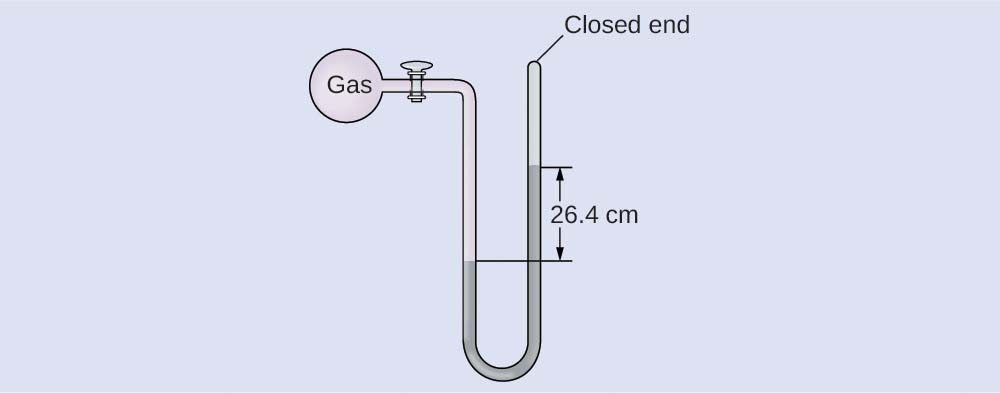#### Solution

The pressure of the gas is equal to a column of mercury of height 26.4 cm. (The pressure at the bottom horizontal line is equal on both sides of the tube. The pressure on the left is due to the gas and the pressure on the right is due to 26.4 cm Hg, or mercury.) We could use the equation p = hρg as in Example 23.2, but it is simpler to just convert between units using Table 23.1.

(a) 26.4cm Hg×10mm Hg1cm Hg×1 torr1mm Hg=264 torr26.4cm Hg×10mm Hg1cm Hg×1 torr1mm Hg=264 torr

(b) 264torr×1atm760torr×101,325 Pa1atm=35,200 Pa264torr×1atm760torr×101,325 Pa1atm=35,200 Pa

(c) 35,200Pa×1 bar100,000Pa=0.352 bar35,200Pa×1 bar100,000Pa=0.352 bar

The pressure of a sample of gas is measured with a closed-end manometer. The liquid in the manometer is mercury. Determine the pressure of the gas in:

(a) torr

(b) Pa

(c) bar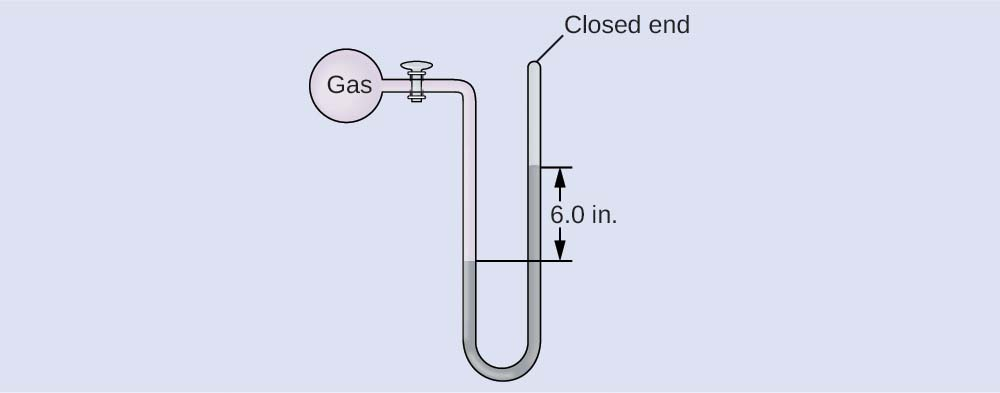(a) ~150 torr; (b) ~20,000 Pa; (c) ~0.20 bar

### Example 23.4

#### Calculation of Pressure Using an Open-End Manometer

The pressure of a sample of gas is measured at sea level with an open-end Hg (mercury) manometer, as shown to the right. Determine the pressure of the gas in:

(a) mm Hg

(b) atm

(c) kPa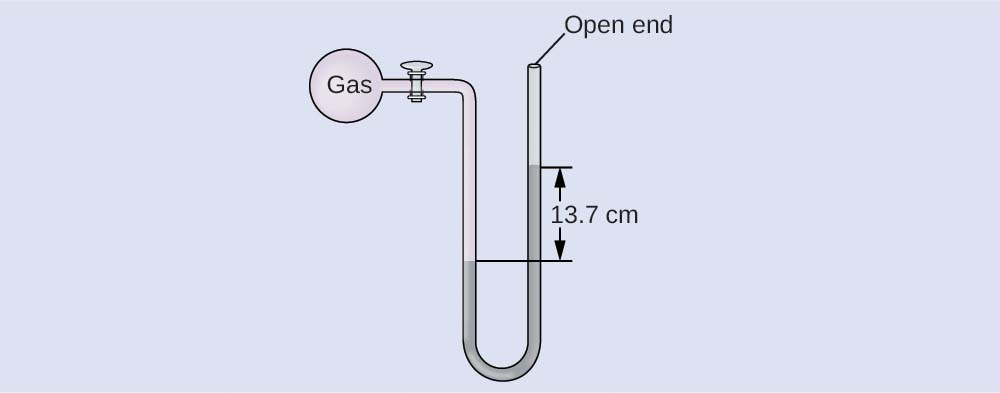#### Solution

The pressure of the gas equals the hydrostatic pressure due to a column of mercury of height 13.7 cm plus the pressure of the atmosphere at sea level. (The pressure at the bottom horizontal line is equal on both sides of the tube. The pressure on the left is due to the gas and the pressure on the right is due to 13.7 cm of Hg plus atmospheric pressure.)

(a) In mm Hg, this is: 137 mm Hg + 760 mm Hg = 897 mm Hg

(b) 897mm Hg×1 atm760mm Hg=1.18 atm897mm Hg×1 atm760mm Hg=1.18 atm

(c) 1.18atm×101.325 kPa1atm=1.20×102kPa1.18atm×101.325 kPa1atm=1.20×102kPa

The pressure of a sample of gas is measured at sea level with an open-end Hg manometer, as shown to the right. Determine the pressure of the gas in:

(a) mm Hg

(b) atm

(c) kPa(a) 642 mm Hg; (b) 0.845 atm; (c) 85.6 kPa

### Chemistry in Everyday Life

#### Measuring Blood Pressure

Blood pressure is measured using a device called a sphygmomanometer (Greek sphygmos = “pulse”). It consists of an inflatable cuff to restrict blood flow, a manometer to measure the pressure, and a method of determining when blood flow begins and when it becomes impeded (Figure 23.5). Since its invention in 1881, it has been an essential medical device. There are many types of sphygmomanometers: manual ones that require a stethoscope and are used by medical professionals; mercury ones, used when the most accuracy is required; less accurate mechanical ones; and digital ones that can be used with little training but that have limitations. When using a sphygmomanometer, the cuff is placed around the upper arm and inflated until blood flow is completely blocked, then slowly released. As the heart beats, blood forced through the arteries causes a rise in pressure. This rise in pressure at which blood flow begins is the systolic pressure—the peak pressure in the cardiac cycle. When the cuff’s pressure equals the arterial systolic pressure, blood flows past the cuff, creating audible sounds that can be heard using a stethoscope. This is followed by a decrease in pressure as the heart’s ventricles prepare for another beat. As cuff pressure continues to decrease, eventually sound is no longer heard; this is the diastolic pressure—the lowest pressure (resting phase) in the cardiac cycle. Blood pressure units from a sphygmomanometer are in terms of millimeters of mercury (mm Hg).

Figure 23.5

(a) A medical technician prepares to measure a patient’s blood pressure with a sphygmomanometer. (b) A typical sphygmomanometer uses a valved rubber bulb to inflate the cuff and a diaphragm gauge to measure pressure. (credit a: modification of work by Master Sgt. Jeffrey Allen)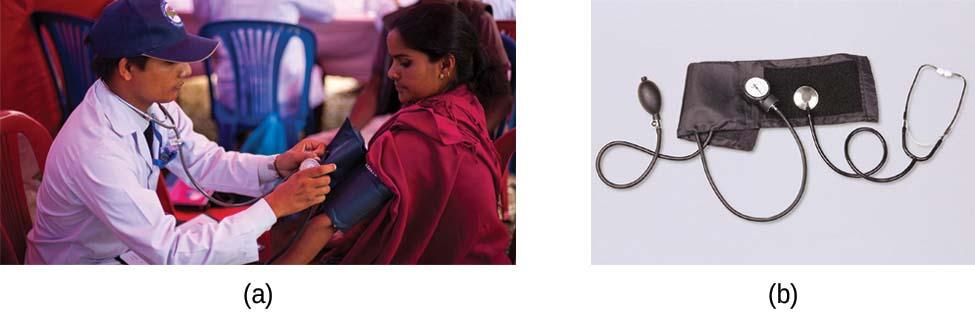### How Sciences Interconnect

#### Meteorology, Climatology, and Atmospheric Science

Throughout the ages, people have observed clouds, winds, and precipitation, trying to discern patterns and make predictions: when it is best to plant and harvest; whether it is safe to set out on a sea voyage; and much more. We now face complex weather and atmosphere-related challenges that will have a major impact on our civilization and the ecosystem. Several different scientific disciplines use chemical principles to help us better understand weather, the atmosphere, and climate. These are meteorology, climatology, and atmospheric science. Meteorology is the study of the atmosphere, atmospheric phenomena, and atmospheric effects on earth’s weather. Meteorologists seek to understand and predict the weather in the short term, which can save lives and benefit the economy. Weather forecasts (Figure 23.6) are the result of thousands of measurements of air pressure, temperature, and the like, which are compiled, modeled, and analyzed in weather centers worldwide.

Figure 23.6

Meteorologists use weather maps to describe and predict weather. Regions of high (H) and low (L) pressure have large effects on weather conditions. The gray lines represent locations of constant pressure known as isobars. (credit: modification of work by National Oceanic and Atmospheric Administration)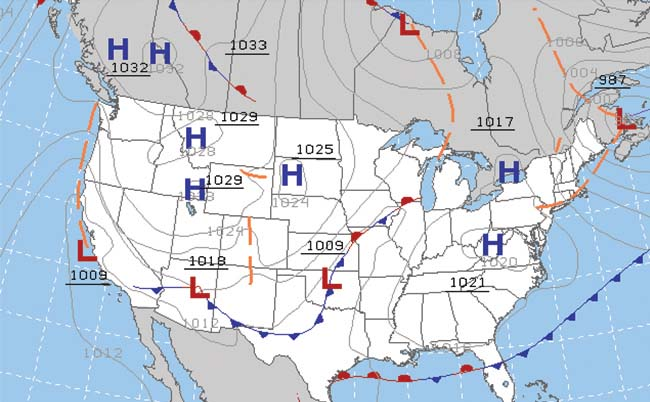In terms of weather, low-pressure systems occur when the earth’s surface atmospheric pressure is lower than the surrounding environment: Moist air rises and condenses, producing clouds. Movement of moisture and air within various weather fronts instigates most weather events.

The atmosphere is the gaseous layer that surrounds a planet. Earth’s atmosphere, which is roughly 100–125 km thick, consists of roughly 78.1% nitrogen and 21.0% oxygen, and can be subdivided further into the regions shown in Figure 23.7: the exosphere (furthest from earth, > 700 km above sea level), the thermosphere (80–700 km), the mesosphere (50–80 km), the stratosphere (second lowest level of our atmosphere, 12–50 km above sea level), and the troposphere (up to 12 km above sea level, roughly 80% of the earth’s atmosphere by mass and the layer where most weather events originate). As you go higher in the troposphere, air density and temperature both decrease.

Figure 23.7

Earth’s atmosphere has five layers: the troposphere, the stratosphere, the mesosphere, the thermosphere, and the exosphere.Climatology is the study of the climate, averaged weather conditions over long time periods, using atmospheric data. However, climatologists study patterns and effects that occur over decades, centuries, and millennia, rather than shorter time frames of hours, days, and weeks like meteorologists. Atmospheric science is an even broader field, combining meteorology, climatology, and other scientific disciplines that study the atmosphere.

Supplemental exercises are available if you would like more practice with these concepts.

## 23.2 Relating Pressure, Volume, Amount, and Temperature: The Ideal Gas Law

### Learning Objectives

By the end of this section, you will be able to:

• Identify the mathematical relationships between the various properties of gases

During the seventeenth and especially eighteenth centuries, driven both by a desire to understand nature and a quest to make balloons in which they could fly (Figure 23.8), a number of scientists established the relationships between the macroscopic physical properties of gases, that is, pressure, volume, temperature, and amount of gas. Although their measurements were not precise by today’s standards, they were able to determine the mathematical relationships between pairs of these variables (e.g., pressure and temperature, pressure and volume) that hold for an ideal gas—a hypothetical construct that real gases approximate under certain conditions. Eventually, these individual laws were combined into a single equation—the ideal gas law—that relates gas quantities for gases and is quite accurate for low pressures and moderate temperatures. We will consider the key developments in individual relationships (for pedagogical reasons not quite in historical order), then put them together in the ideal gas law.

Figure 23.8

In 1783, the first (a) hydrogen-filled balloon flight, (b) manned hot air balloon flight, and (c) manned hydrogen-filled balloon flight occurred. When the hydrogen-filled balloon depicted in (a) landed, the frightened villagers of Gonesse reportedly destroyed it with pitchforks and knives. The launch of the latter was reportedly viewed by 400,000 people in Paris.### Pressure and Temperature: Amontons’s Law

Imagine filling a rigid container attached to a pressure gauge with gas and then sealing the container so that no gas may escape. If the container is cooled, the gas inside likewise gets colder and its pressure is observed to decrease. Since the container is rigid and tightly sealed, both the volume and number of moles of gas remain constant. If we heat the sphere, the gas inside gets hotter (Figure 23.9) and the pressure increases.

Figure 23.9

The effect of temperature on gas pressure: When the hot plate is off, the pressure of the gas in the sphere is relatively low. As the gas is heated, the pressure of the gas in the sphere increases.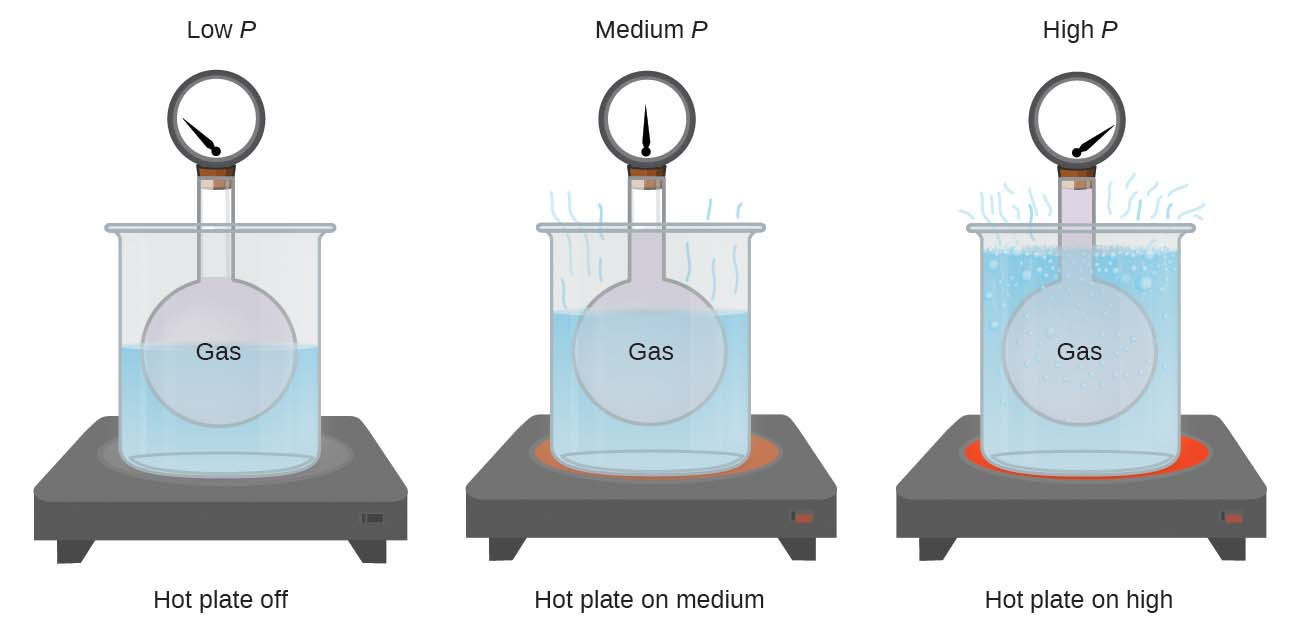This relationship between temperature and pressure is observed for any sample of gas confined to a constant volume. An example of experimental pressure-temperature data is shown for a sample of air under these conditions in Figure 23.10. We find that temperature and pressure are linearly related, and if the temperature is on the kelvin scale, then P and T are directly proportional (again, when volume and moles of gas are held constant); if the temperature on the kelvin scale increases by a certain factor, the gas pressure increases by the same factor.

Figure 23.10

For a constant volume and amount of air, the pressure and temperature are directly proportional, provided the temperature is in kelvin. (Measurements cannot be made at lower temperatures because of the condensation of the gas.) When this line is extrapolated to lower pressures, it reaches a pressure of 0 at –273 °C, which is 0 on the kelvin scale and the lowest possible temperature, called absolute zero.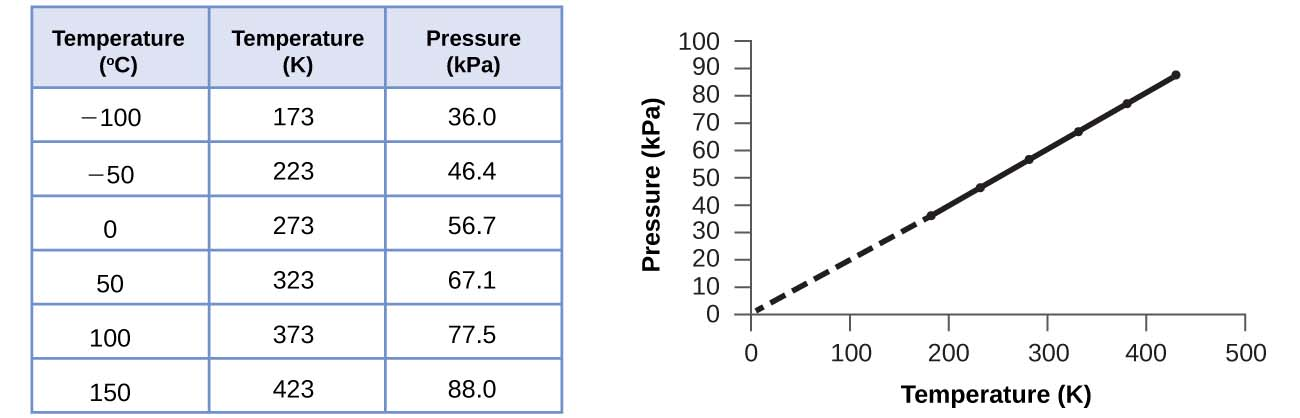Guillaume Amontons was the first to empirically establish the relationship between the pressure and the temperature of a gas (~1700), and Joseph Louis Gay-Lussac determined the relationship more precisely (~1800). Because of this, the P-T relationship for gases is known as either Amontons’s law or Gay-Lussac’s law. Under either name, it states that the pressure of a given amount of gas is directly proportional to its temperature on the kelvin scale when the volume is held constant. Mathematically, this can be written:

P∝TorP=constant×TorP=k×TP∝TorP=constant×TorP=k×T

where ∝ means “is proportional to,” and k is a proportionality constant that depends on the identity, amount, and volume of the gas.

For a confined, constant volume of gas, the ratio PTPT is therefore constant (i.e., PT=kPT=k). If the gas is initially in “Condition 1” (with P = P1 and T = T1), and then changes to “Condition 2” (with P = P2 and T = T2), we have that P1T1=kP1T1=k and P2T2=k,P2T2=k, which reduces to P1T1=P2T2.P1T1=P2T2. This equation is useful for pressure-temperature calculations for a confined gas at constant volume. Note that temperatures must be on the kelvin scale for any gas law calculations (0 on the kelvin scale and the lowest possible temperature is called absolute zero). (Also note that there are at least three ways we can describe how the pressure of a gas changes as its temperature changes: We can use a table of values, a graph, or a mathematical equation.)

### Example 23.5

#### Predicting Change in Pressure with Temperature

A can of hair spray is used until it is empty except for the propellant, isobutane gas.

(a) On the can is the warning “Store only at temperatures below 120 °F (48.8 °C). Do not incinerate.” Why?

(b) The gas in the can is initially at 24 °C and 360 kPa, and the can has a volume of 350 mL. If the can is left in a car that reaches 50 °C on a hot day, what is the new pressure in the can?

#### Solution

(a) The can contains an amount of isobutane gas at a constant volume, so if the temperature is increased by heating, the pressure will increase proportionately. High temperature could lead to high pressure, causing the can to burst. (Also, isobutane is combustible, so incineration could cause the can to explode.)

(b) We are looking for a pressure change due to a temperature change at constant volume, so we will use Amontons’s/Gay-Lussac’s law. Taking P1 and T1 as the initial values, T2 as the temperature where the pressure is unknown and P2 as the unknown pressure, and converting °C to K, we have:

P1T1=P2T2which means that360kPa297K=P2323KP1T1=P2T2which means that360kPa297K=P2323K

Rearranging and solving gives: P2=360kPa×323K297K=390kPaP2=360kPa×323K297K=390kPa

A sample of nitrogen, N2, occupies 45.0 mL at 27 °C and 600 torr. What pressure will it have if cooled to –73 °C while the volume remains constant?

400 torr

### Volume and Temperature: Charles’s Law

If we fill a balloon with air and seal it, the balloon contains a specific amount of air at atmospheric pressure, let’s say 1 atm. If we put the balloon in a refrigerator, the gas inside gets cold and the balloon shrinks (although both the amount of gas and its pressure remain constant). If we make the balloon very cold, it will shrink a great deal, and it expands again when it warms up.

These examples of the effect of temperature on the volume of a given amount of a confined gas at constant pressure are true in general: The volume increases as the temperature increases, and decreases as the temperature decreases. Volume-temperature data for a 1-mole sample of methane gas at 1 atm are listed and graphed in Figure 23.11.

Figure 23.11

The volume and temperature are linearly related for 1 mole of methane gas at a constant pressure of 1 atm. If the temperature is in kelvin, volume and temperature are directly proportional. The line stops at 111 K because methane liquefies at this temperature; when extrapolated, it intersects the graph’s origin, representing a temperature of absolute zero.The relationship between the volume and temperature of a given amount of gas at constant pressure is known as Charles’s law in recognition of the French scientist and balloon flight pioneer Jacques Alexandre César Charles. Charles’s law states that the volume of a given amount of gas is directly proportional to its temperature on the kelvin scale when the pressure is held constant.

Mathematically, this can be written as:

VαTorV=constant·TorV=k·TorV1/T1=V2/T2VαTorV=constant·TorV=k·TorV1/T1=V2/T2

with k being a proportionality constant that depends on the amount and pressure of the gas.

For a confined, constant pressure gas sample, VTVT is constant (i.e., the ratio = k), and as seen with the P-T relationship, this leads to another form of Charles’s law: V1T1=V2T2.V1T1=V2T2.

### Example 23.6

#### Predicting Change in Volume with Temperature

A sample of carbon dioxide, CO2, occupies 0.300 L at 10 °C and 750 torr. What volume will the gas have at 30 °C and 750 torr?

#### Solution

Because we are looking for the volume change caused by a temperature change at constant pressure, this is a job for Charles’s law. Taking V1 and T1 as the initial values, T2 as the temperature at which the volume is unknown and V2 as the unknown volume, and converting °C into K we have:
V1T1=V2T2which means that0.300L283K=V2303KV1T1=V2T2which means that0.300L283K=V2303K

Rearranging and solving gives: V2=0.300L×303K283K=0.321LV2=0.300L×303K283K=0.321L

This answer supports our expectation from Charles’s law, namely, that raising the gas temperature (from 283 K to 303 K) at a constant pressure will yield an increase in its volume (from 0.300 L to 0.321 L).

A sample of oxygen, O2, occupies 32.2 mL at 30 °C and 452 torr. What volume will it occupy at –70 °C and the same pressure?

21.6 mL

### Example 23.7

#### Measuring Temperature with a Volume Change

Temperature is sometimes measured with a gas thermometer by observing the change in the volume of the gas as the temperature changes at constant pressure. The hydrogen in a particular hydrogen gas thermometer has a volume of 150.0 cm3 when immersed in a mixture of ice and water (0.00 °C). When immersed in boiling liquid ammonia, the volume of the hydrogen, at the same pressure, is 131.7 cm3. Find the temperature of boiling ammonia on the kelvin and Celsius scales.

#### Solution

A volume change caused by a temperature change at constant pressure means we should use Charles’s law. Taking V1 and T1 as the initial values, T2 as the temperature at which the volume is unknown and V2 as the unknown volume, and converting °C into K we have:
V1T1=V2T2which means that150.0cm3273.15K=131.7cm3T2V1T1=V2T2which means that150.0cm3273.15K=131.7cm3T2

Rearrangement gives T2=131.7cm3×273.15K150.0cm3=239.8KT2=131.7cm3×273.15K150.0cm3=239.8K

Subtracting 273.15 from 239.8 K, we find that the temperature of the boiling ammonia on the Celsius scale is –33.4 °C.

What is the volume of a sample of ethane at 467 K and 1.1 atm if it occupies 405 mL at 298 K and 1.1 atm?

635 mL

### Volume and Pressure: Boyle’s Law

If we partially fill an airtight syringe with air, the syringe contains a specific amount of air at constant temperature, say 25 °C. If we slowly push in the plunger while keeping temperature constant, the gas in the syringe is compressed into a smaller volume and its pressure increases; if we pull out the plunger, the volume increases and the pressure decreases. This example of the effect of volume on the pressure of a given amount of a confined gas is true in general. Decreasing the volume of a contained gas will increase its pressure, and increasing its volume will decrease its pressure. In fact, if the volume increases by a certain factor, the pressure decreases by the same factor, and vice versa. Volume-pressure data for an air sample at room temperature are graphed in Figure 23.12.

Figure 23.12

When a gas occupies a smaller volume, it exerts a higher pressure; when it occupies a larger volume, it exerts a lower pressure (assuming the amount of gas and the temperature do not change). Since P and V are inversely proportional, a graph of 1P1P vs. V is linear.Unlike the P-T and V-T relationships, pressure and volume are not directly proportional to each other. Instead, P and V exhibit inverse proportionality: Increasing the pressure results in a decrease of the volume of the gas. Mathematically this can be written:

Pα1/VorP=k·1/VorP·V=korP1V1=P2V2Pα1/VorP=k·1/VorP·V=korP1V1=P2V2

with k being a constant. Graphically, this relationship is shown by the straight line that results when plotting the inverse of the pressure (1P)(1P) versus the volume (V), or the inverse of volume (1V)(1V) versus the pressure (P). Graphs with curved lines are difficult to read accurately at low or high values of the variables, and they are more difficult to use in fitting theoretical equations and parameters to experimental data. For those reasons, scientists often try to find a way to “linearize” their data. If we plot P versus V, we obtain a hyperbola (see Figure 23.13).

Figure 23.13

The relationship between pressure and volume is inversely proportional. (a) The graph of P vs. V is a hyperbola, whereas (b) the graph of (1P)(1P) vs. V is linear.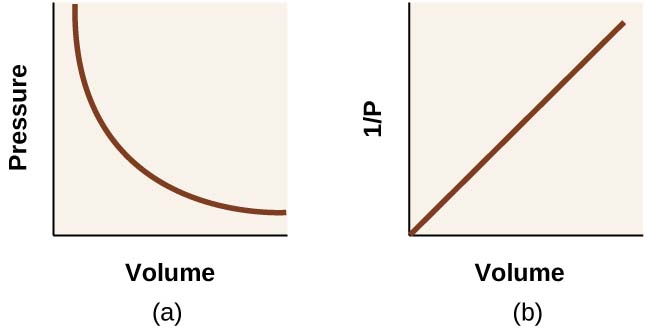The relationship between the volume and pressure of a given amount of gas at constant temperature was first published by the English natural philosopher Robert Boyle over 300 years ago. It is summarized in the statement now known as Boyle’s law: The volume of a given amount of gas held at constant temperature is inversely proportional to the pressure under which it is measured.

### Example 23.8

#### Volume of a Gas Sample

The sample of gas in Figure 23.12 has a volume of 15.0 mL at a pressure of 13.0 psi. Determine the pressure of the gas at a volume of 7.5 mL, using:

(a) the P-V graph in Figure 23.12

(b) the 1P1P vs. V graph in Figure 23.12

(c) the Boyle’s law equation

Comment on the likely accuracy of each method.

#### Solution

(a) Estimating from the P-V graph gives a value for P somewhere around 27 psi.

(b) Estimating from the 1P1P versus V graph give a value of about 26 psi.

(c) From Boyle’s law, we know that the product of pressure and volume (PV) for a given sample of gas at a constant temperature is always equal to the same value. Therefore we have P1V1 = k and P2V2 = k which means that P1V1 = P2V2.

Using P1 and V1 as the known values 13.0 psi and 15.0 mL, P2 as the pressure at which the volume is unknown, and V2 as the unknown volume, we have:

P1V1=P2V2or13.0psi×15.0mL=P2×7.5mLP1V1=P2V2or13.0psi×15.0mL=P2×7.5mL

Solving:

P2=13.0psi×15.0mL7.5mL=26psiP2=13.0psi×15.0mL7.5mL=26psi

It was more difficult to estimate well from the P-V graph, so (a) is likely more inaccurate than (b) or (c). The calculation will be as accurate as the equation and measurements allow.

The sample of gas in Figure 23.12 has a volume of 30.0 mL at a pressure of 6.5 psi. Determine the volume of the gas at a pressure of 11.0 psi, using:

(a) the P-V graph in Figure 23.12

(b) the 1P1P vs. V graph in Figure 23.12

(c) the Boyle’s law equation

Comment on the likely accuracy of each method.

(a) about 17–18 mL; (b) ~18 mL; (c) 17.7 mL; it was more difficult to estimate well from the P-V graph, so (a) is likely more inaccurate than (b); the calculation will be as accurate as the equation and measurements allow

### Chemistry in Everyday Life

#### Breathing and Boyle’s Law

Figure 23.14

Breathing occurs because expanding and contracting lung volume creates small pressure differences between your lungs and your surroundings, causing air to be drawn into and forced out of your lungs.### Moles of Gas and Volume: Avogadro’s Law

The Italian scientist Amedeo Avogadro advanced a hypothesis in 1811 to account for the behavior of gases, stating that equal volumes of all gases, measured under the same conditions of temperature and pressure, contain the same number of molecules. Over time, this relationship was supported by many experimental observations as expressed by Avogadro’s law: For a confined gas, the volume (V) and number of moles (n) are directly proportional if the pressure and temperature both remain constant.

In equation form, this is written as:

V∝norV=k×norV1n1=V2n2V∝norV=k×norV1n1=V2n2

Mathematical relationships can also be determined for the other variable pairs, such as P versus n, and n versus T.